Maths-
General
Easy

Question

# The simple interest on a sum of money at the end of 3 years is of the sum itself. What rate percent was charged?Hint:

## The correct answer is: 6.25%

### Complete step by step solution:Let the sum of money = PIt is given that SI is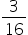times the sum itself =P.We calculate simple interest by the formula,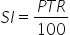where P is Principal amount, T is number of years and R is rate of interestHere, we have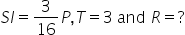On substituting the known values we get,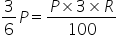On further simplifications, we have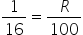.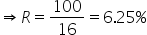#### With Turito Foundation.#### Get an Expert Advice From Turito.